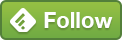## May 26 Analyze Day Of Week GA Data via R Programming [Geom_Boxplots]

So here's a the first [of many, hopefully] blog pots that I'll write as I learn stuff in R Programming. This is basic as I'm just working my way through a R programming course http://analyticslog.com/blog/learning-r-programming-via-udemy-course

Of course, nothing here [on my R posts] is original but rather based on what I understand from other's articles (Stackoverflow, Tim Wilson, Ryan Praskievicz, R-Bloggers, Reddit/r/rstudio). Wherever I can [and will try to keep a tab of specific pages], will post links to give full credit to the actual code. Unfortunately, I can't recall if the this particular code was on StackOverflow before being modified by me.

## Analyze Google Analytics day of week data in R Studio via Geom_boxplots

Let's start.

We are trying to find out how does traffic to the website vary by day of the week. More than knowing just the absolute numbers, we'd also like to know about the median, quartiles, range etc.

Here's the code first.

```library(googleAnalyticsR)
ga_auth()
#replace viewID 1234567 with your Google Analytics view ID
gadata <- google_analytics(viewId = 1234567,
date_range = c(Sys.Date()-100, Sys.Date()-1),
metrics = c("users", "sessions", "pageviews"),
dimensions = c("date", "dayofWeek"),
anti_sample = TRUE)

head(gadata)

str(gadata)

#ggplot
boxplotchart <- ggplot(gadata, aes(x=dayofWeek, y = sessions)) +
geom_boxplot()

boxplotchart +
ylab("Sessions on that day") +
xlab("Day of Week; 0 = Sun, 6 = Sat")

```

For n00bs like me, here's what it goes:

library(googleAnalyticsR) loads the GA library.

ga_aut() creates an authorization token that needs to be entered into the console window before beginning to pull data.

`date_range = c(Sys.Date()-100, Sys.Date()-1) `

tells R to pull for last 100 days' data.

```metrics = c("users", "sessions", "pageviews"),

dimensions = c("date", "dayofWeek"),```

tells us what do we want to pull from GA. Here's the full list of values for GA API:

https://developers.google.com/analytics/devguides/reporting/core/dimsmets#cats=time

The head(gadata) shows the first 6 rows and what the values look like

The str(gadata) tells us the structure of the data [str = structure in R, not string]. Below, we can see that there are 100 observations with 5 variables [users, sessions, pageviews, data, dayofWeek] with values for the variables [Dimensions and Metrics]

```boxplotchart <- ggplot(gadata, aes(x=dayofWeek, y = sessions)) +
geom_boxplot() ```

creates the boxplot where the X axis is day of week and y axis is sessions.

```boxplotchart +
ylab("Sessions on that day") +
xlab("Day of Week; 0 = Sun, 6 = Sat") ```

creates a custom label for x and y axis.

So, what can we know from the boxplot.

Looks like traffic to my blog is heavy [relatively] during weekdays compared to weekends. You can see an inverted arc. Fri, Sat, Sun is when traffic starts dying out.

The horizontal line in between the boxes is the median value. It's again highest on Tuesdays [around 52]

On Tuesdays, 50% of the values are between ~45 and 58.

The highest peak for Tuesday traffic has been 68 sessions.

Looks like I should pushing more content/promoting it on weekdays!

More details on reading boxplots:

http://www.whatissixsigma.net/box-plot-diagram-to-identify-outliers/